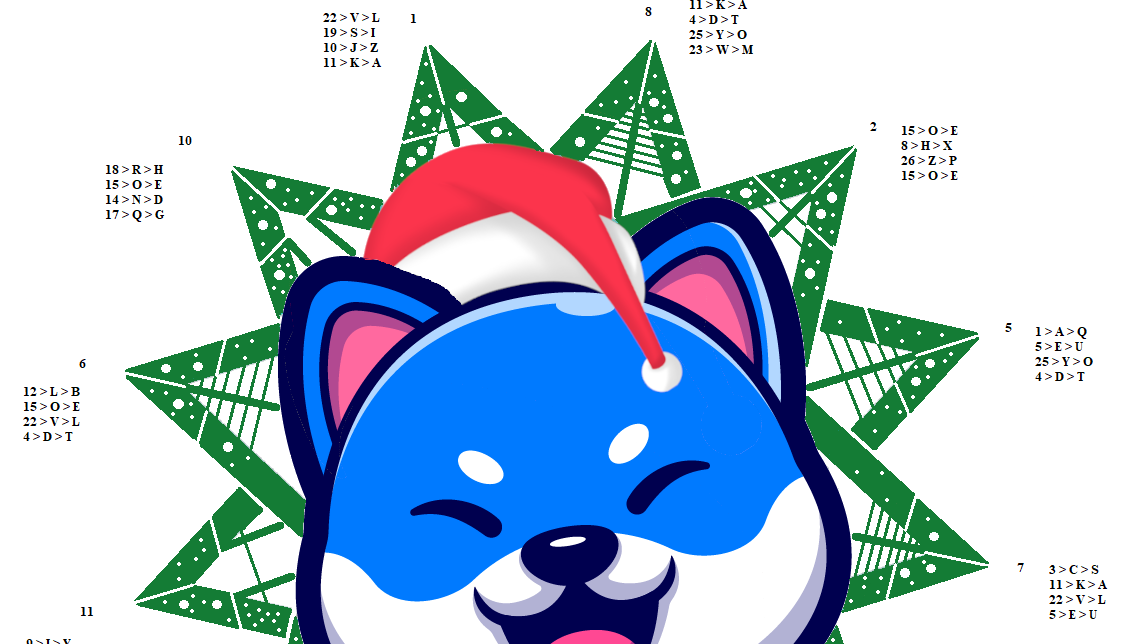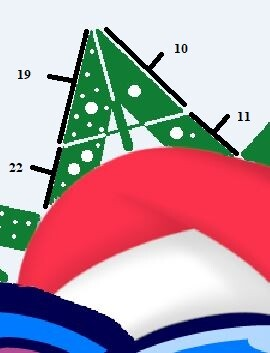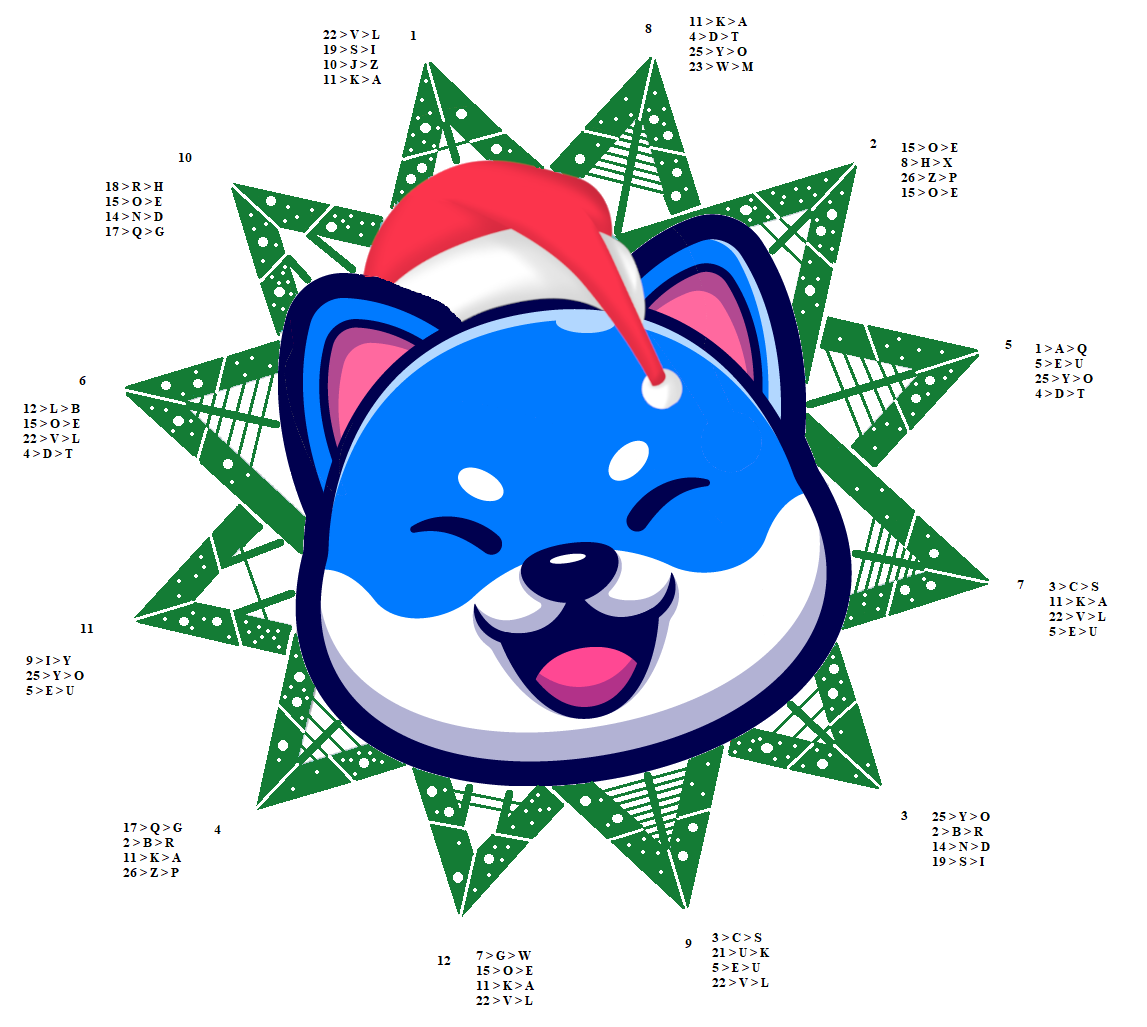# Puzzle #9 Solution

By shuttlecock | Shuttlecock Puzzles | 26 Dec 2021

The image in concern is a logo on VINU placed on a 12-pointed star (dodecagram). Each point of the star contains lines of various thicknesses, and circles of various sizes. The challenge is to extract information about the 12-word mnemonic phrase for the Vite wallet.

Let us take a closer look at the image.Title: VINU X+MAS ROUND THE CLOCK

Each of the 12 points of the star contains information about one word of the 12-word mnemonic phrase, as well as its position within that phrase.

Here is how we solve the puzzle.

First, let us focus on the lines. Each point of the star contains thick and thin lines. The thick lines = 10, while the thin lines = 1. So, the following image (which contains 1 thick line and 1 thin line) = 11.Proceed to do the same for the rest of the image, and you will get this result:Notice that the numbers are from 11 to 22. These numbers are actually 1 to 12, but displaced by 10. We can easily infer this, or we can get a hint from the title “X+MAS”. “X” is the Roman numeral for 10. And “+” is, well, addition. So, what we need to do is to subtract 10 from each number from 11 to 22. And we get the following:Next, we move to the circles. We see that there are big and small circles. Big circle = 10, small circle = 1. Now, we also see that each point of the star is split into 4 parts. Each of these parts contains information about a letter (of a mnemonic word).

Starting with the circles, we get something like this:These numbers refer to the position of the letter in the alphabetic. So, A=1, Z=26. This is a simple substitution cipher, sometimes known as “A1Z26”. So, what we get is something like this:

10 > J

11 > K

19 > S

22 > V

How do we make sense of these letters? We return to the earlier clue “X+MAS” – which suggests that the letters may be displaced by +10 again. So, what we need to do is to subtract 10 (move backwards by 10) from each letter. This is another substitution cipher, sometimes known as the shift cipher And we get the following:

J – 10 > Z

K – 10 > A

S – 10 > I

V – 10 > L

Now, we need to arrange these letters. A second clue, hidden in the title, comes into play – “ROUND THE CLOCK”. This suggests that the letters should be arranged in a clockwise manner. So, we get this:

22 > V > L

19 > S > I

10 > J > Z

11 > K > A

Now, we search for these letters on the BIP-39 word list. The BIP-39 list is a list of 2048 words. All the words in seed phrases are taken from this list. An interesting feature of the BIP-39 list is that the first 4 letters are unique to each word within the list. That is, there are no two words in this list that have the same first 4 letters. This means that if you have the first four letters of the word, you effectively know the word (by referring to the BIP-39 list).

When we search for “LIZA”, we get “LIZARD” as the only result.

Do the same for the other columns, and you will eventually get the following:

1          lizard

2          expect

3          ordinary

4          grape

5          quote

6          belt

7          salute

8          atom

9          skull

10        hedgehog

11        you

12        wealthAnd the puzzle is solved!shuttlecock